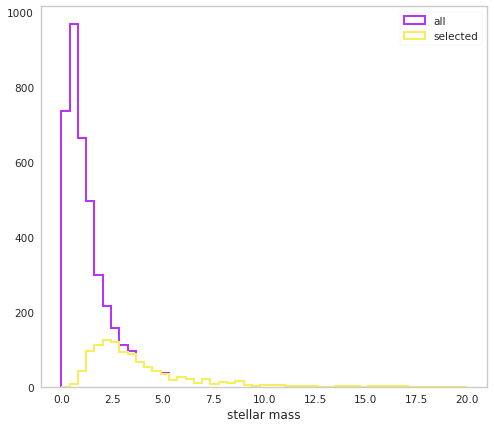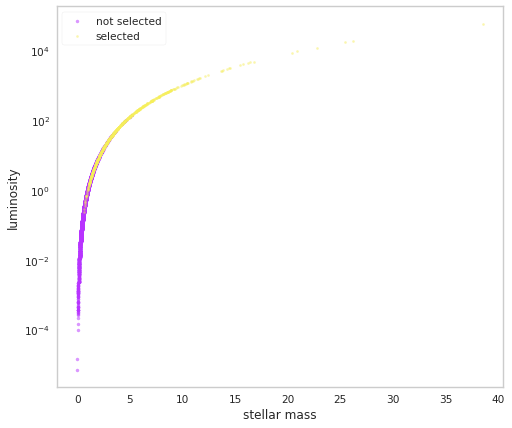# Stellar Mass-Luminosity Bias

Suppose that stars have a mass-luminosity relationship such that $$L \propto M^3$$. If we have a flux-limited survey, it will bias us towards observing more massive stars which are not representative of the full mass distribution. Let’s see how to set this up in popsynth.

## Setup the problem

First, we will assume that we have some initial mass function (IMF) for our stars that describes how there masses are distributed. For simplicity, we will assume that this IMF is just a log-normal distribution:

:

%matplotlib inline

import matplotlib.pyplot as plt
from jupyterthemes import jtplot

jtplot.style(context="notebook", fscale=1, grid=False)
purple = "#B833FF"
yellow = "#F6EF5B"

import networkx as nx
import numpy as np
import warnings

warnings.simplefilter("ignore")

import popsynth

# create a sampler for mass
# we do not directly observe the mass as it is a latent quantity

initial_mass_function = popsynth.LogNormalAuxSampler(name="mass", observed = False)


We now assume the dependent variable is the luminosity, so we need a DerivedLumAuxSampler that generates luminosities given a mass:

:

class MassLuminosityRelation(popsynth.DerivedLumAuxSampler):
_auxiliary_sampler_name = "MassLuminosityRelation"

def __init__(self, mu=0.0, tau=1.0, sigma=1):
# this time set observed=True
super(MassLuminosityRelation, self).__init__("mass_lum_relation", uses_distance=False)

def true_sampler(self, size):

# the secondary quantity is mass

mass = self._secondary_samplers["mass"].true_values

# we will store the log of mass cubed
self._true_values = 3 * np.log10(mass)

def compute_luminosity(self):
# compute the luminosity
# from the relation
return np.power(10., self._true_values)

luminosity = MassLuminosityRelation()


Now we can put everything together. First, we need to assign mass as a secondary quantity to the luminosity

:

luminosity.set_secondary_sampler(initial_mass_function)


Finally, we will use a simple spherical geometry to hold our stars. We will also put a hard flux limit on our survey to simulate a flux-limited catalog.

:

pop_gen = popsynth.populations.SphericalPopulation(1, r_max=10)

# create the flux selection

flux_selector = popsynth.HardFluxSelection()
flux_selector.boundary = 1e-2
pop_gen.set_flux_selection(flux_selector)

# now add the luminisity sampler



Now let’s draw our survey.

:

pop = pop_gen.draw_survey(flux_sigma=0.5)


We can now look at the distribution of the masses:

:

fig, ax = plt.subplots()

bins = np.linspace(0,20,50)

ax.hist(pop.mass,
bins=bins,
label='all',
color=purple,
histtype="step",
lw=2

)

ax.hist(pop.mass[pop.selection],
bins=bins,
label='selected',
color=yellow,
histtype="step",
lw=2
)

ax.set_xlabel('stellar mass')
ax.legend()

:

<matplotlib.legend.Legend at 0x7feb7ce83250>We can see that indeed our selected masses are biased towards higher values.

Let’s look in the mass-luminostiy plane:

:

fig, ax = plt.subplots()

bins = np.linspace(0,20,50)

ax.scatter(pop.mass[~pop.selection],
pop.luminosities_latent[~pop.selection],
label='not selected',
color=purple,
alpha=0.5,
s=10

)

ax.scatter(pop.mass[pop.selection],
pop.luminosities_latent[pop.selection],
label='selected',
color=yellow,
alpha=0.5,
s=5
)

ax.set_xlabel('stellar mass')
ax.set_ylabel('luminosity')
ax.set_yscale('log')

ax.legend()

:

<matplotlib.legend.Legend at 0x7feb79aa1eb0>